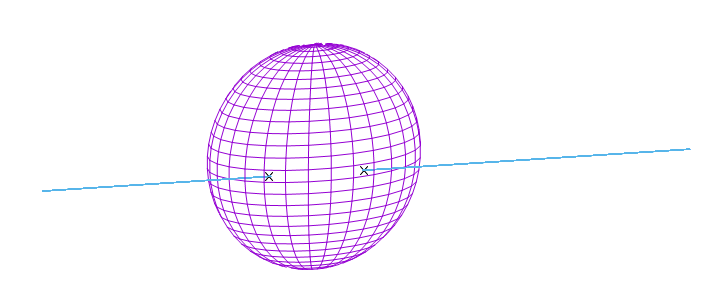## Ray-Sphere IntersectionWhen developing atmospheric scattering shaders, we will need to calculate intersection points between rays and spheres. So here is how we can do that.

Defining a ray
To define a ray, we specify two vectors: One point o, which goes from zero to a point on the ray and another one d, which goes along the ray and defines therefore the ray’s direction. The direction vector is multiplied by an argument t. When varying t, we can reach any point on the ray. Now you might say, that we did not define a ray, but a line. The only difference between rays and lines is, that t has a minimum value for rays. A line goes from -infinity to infinity, while a ray has a starting point and goes to infinity from there. In our use case, that’s not that important tough.$\displaystyle\overrightarrow{x}=\overrightarrow{o}+t\overrightarrow{d}$

Defining a sphere
To define a sphere, we need to specify a center point c and a radius r. All points on a sphere are points, which have a distance to the center point equally to the sphere’s radius. So a sphere’s definition can be:$\displaystyle\|\overrightarrow{x}-\overrightarrow{c}\|=r$ If we can assume, that the center of the sphere is at the origin of our coordinate system, the equation simplifys to$\displaystyle\|\overrightarrow{x}\|=r$, so “all vectors with a certain length r”.

Intersecting both
In our use case, we will have the spheres always centered, so we can use the easier version of the sphere definition. Inserting the ray definition into the sphere definition yields:$\displaystyle\|\overrightarrow{o}+t_{\mathrm{hit}}\overrightarrow{d}\|=r$ I replaced t with$\displaystyle t_{\mathrm{hit}}$ because now it is not a varying parameter anymore. Instead it is a symbol for all values which fulfill this equation (a ray can hit a sphere zero, one or two times).
Image a vector$\displaystyle\overrightarrow{a}\$, then there is:$\displaystyle\|\overrightarrow{a}\|^2 = \|\overrightarrow{a}\|\|\overrightarrow{a}\|$

From the definition of the dot product and$\displaystyle\cos{0}=1 \Rightarrow \overrightarrow{a}\cdot\overrightarrow{a}=\|\overrightarrow{a}\|\|\overrightarrow{a}\|\cos{\alpha} = \|\overrightarrow{a}\|\|\overrightarrow{a}\|$
So there is$\displaystyle\|\overrightarrow{a}\|^2 = \overrightarrow{a}\cdot\overrightarrow{a}$

To apply this to the intersection, we need to square it first:$\displaystyle\|\overrightarrow{o}+t_{\mathrm{hit}}\overrightarrow{d}\|=r \Leftrightarrow r^2 =\|\overrightarrow{o}+t_{\mathrm{hit}}\overrightarrow{d}\|^2$$=(\overrightarrow{o}+t_{\mathrm{hit}}\overrightarrow{d})\cdot(\overrightarrow{o}+t_{\mathrm{hit}}\overrightarrow{d})$$\displaystyle=\overrightarrow{o}^2+2\overrightarrow{o}t_{\mathrm{hit}}\overrightarrow{d}+(t_{\mathrm{hit}}\overrightarrow{d})^2 =\overrightarrow{o}^2+2\overrightarrow{o}\cdot\overrightarrow{d}t_{\mathrm{hit}}+\overrightarrow{d}^2t_{\mathrm{hit}}^2$$\displaystyle\Leftrightarrow\overrightarrow{o}^2+2\overrightarrow{o}\cdot\overrightarrow{d}t_{\mathrm{hit}}+\overrightarrow{d}^2t_{\mathrm{hit}}^2-r^2=0$$\displaystyle\Leftrightarrow \overrightarrow{d}^2t_{\mathrm{hit}}^2 +2\overrightarrow{o}\cdot\overrightarrow{d}t_{\mathrm{hit}}+\overrightarrow{o}^2-r^2=0$$\displaystyle\Leftrightarrow t_{\mathrm{hit}}^2 +\frac{2\overrightarrow{o}\cdot\overrightarrow{d}}{\overrightarrow{d}^2}t_{\mathrm{hit}}+\frac{\overrightarrow{o}^2-r^2}{\overrightarrow{d}^2}=0$

To make the calculation easier we assume, that$\displaystyle\overrightarrow{d}$ is normalized:$\displaystyle\|\overrightarrow{d}\|=1 \Rightarrow \overrightarrow{d}^2 = \overrightarrow{d}\cdot\overrightarrow{d} = \|\overrightarrow{d}\|\|\overrightarrow{d}\| = 1*1 = 1$ So the denominators above are one and are left out.

Now we use the reduced quadratic formula to solve the equation for$\displaystyle t_{\mathrm{hit}}$:$\displaystyle p=2\overrightarrow{o}\overrightarrow{d}, q=\overrightarrow{o}^2-r^2$
The discriminant is$\displaystyle D=\left(\frac{p}{2}\right)^2-q$$\displaystyle t_{\mathrm{hit}}=-\frac{p}{2}\pm\sqrt{D}$$\displaystyle=-\frac{2\overrightarrow{o}\overrightarrow{d}}{2}\pm\sqrt{\left(\frac{2\overrightarrow{o}\overrightarrow{d}}{2}\right)^2-\overrightarrow{o}^2+r^2}$$\displaystyle= -\overrightarrow{o}\overrightarrow{d}\pm\sqrt{\left(\overrightarrow{o}\overrightarrow{d}\right)^2-\overrightarrow{o}^2+r^2}$
So the cheapest way to calculate the intersection point is:$\displaystyle t_{\mathrm{hit}}=-a\pm\sqrt{a^2-\overrightarrow{o}^2+r^2}$ with$a=\overrightarrow{o}\cdot\overrightarrow{d}$

Keep in mind, that$\overrightarrow{d}$ has to be normalized!

## Numerical Integration

To describe atmospheric scattering, we will use several equations with integrals which can’t be solved analytically – so we will make use of middle Riemann sums to approximate those.

Image a function f which can’t be integrated analytically. It might have a function graph like this:In this case it is the graph for$f(x)=e^{-x}$, which is important for atmospheric scattering. Of course, we can integrate it directly, but we will use this function anyway, so that we can compare our approximation with the direct result.

Let’s say, we want to integrate it from x=1 to x=2:To calculate this integral, we will divide the complete integral into n intervals and approximate each part with a rectangle, for example n=3:When the bounds are a und b, each interval has a width of:$\displaystyle \mathrm{d}=\frac{b-a}{n}=\frac{2-1}{3}=\frac{1}{3}$

Next we need the height of each rectangle. Here there are several possibilities to choose from, we use the value of our function in the middle of each interval (that’s why it is called middle sum):Now we can calculate the area of each rectangle:$\displaystyle A_i = \mathrm{d}\times f(a+(i+\frac{1}{2})\mathrm{d})$

i a+(i+0.5)d f(a+(i+0.5)d) Ai
0 1.16666666666667 0.311403223914598 0.103801074638199
1 1.5 0.22313016014843 0.074376720049477
2 1.83333333333333 0.159879746079694 0.053293248693231$\displaystyle\sum A_i = 0.231471043380907$

Let’s check:$\displaystyle\int_1^2 e^{-x}= \left[ -e^{-x} \right]_1^2 = -e^{-2}+e^{-1}=\frac{1}{e}-\frac{1}{e^2}=\frac{e}{e^2}-\frac{1}{e^2}$$\displaystyle=\frac{e-1}{e^2}\approx 0.23254415793483$

In this case there is an error of 0.46606%, pretty good for just using three sample intervals. When using more samples, the approximation gets more accurate, here are the results when using 3, 10, 50 and 500 samples:

n Approximation Error
3 0.231471043380907 0.461467 %
10 0.232447292788817 0.041655 %
50 0.232540282244081 0.001667 %
500 0.232544119177475 0.000017 %

There is a lot of theory for quantifying the error, but I will omit it here. All we will need is the following equation for numeric integration by middle Riemann sums:$\displaystyle\int_a^b f(x)\,dx \approx \frac{b-a}{n}\sum_{i=0}^{n-1} f\left(a+\left(\frac{1}{2}+i\right)\frac{b-a}{n}\right)$